The Surfaces of Gerhard Miehlich

Consider a sphere, S, with radius c>0, and with center at point C. Let L be a straight line in space. The locus of points in space which are equidistant from the line and the sphere is a "surface of Gerhard Miehlich." For a point P, let b denote the (non-negative) distance from P to L. Let a denote the (non-negative) distance from P to the point C. The distance from P to the sphere is a - c, if P is outside the sphere, c - a, if P is inside the sphere, or 0, if P is on the sphere. So, the locus of equidistant points is defined by b = |a - c|. If C is not on L, then there's a coordinate system (x,y,z) so that the line L is the z-axis, the center of the sphere is C = (1,0,0), and the equation of the surface is:
(1 - 2x + z2 - c2)2 - 4c2(x2 + y2) = 0.
If C is on L, there are coordinates so that L is the z-axis and C = (0,0,0), and the implicit equation becomes
(z2 - c2)2 - 4c2(x2 + y2) = 0.

Although points which are equidistant from L and S must satisfy the implicit equation, some of the following cases will show that there may be solutions of the implicit equation which are not equidistant points.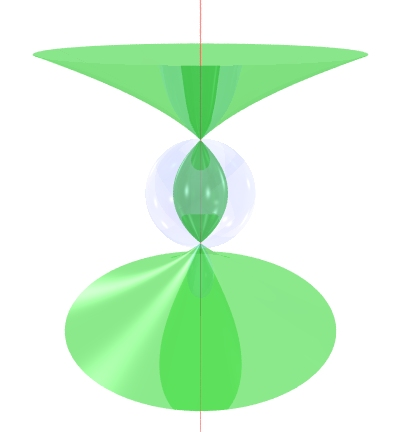In this picture (and the following pictures), S is the transparent blue sphere, L is the red line, and the surface of equidistant points is green. This surface is the rotationally symmetric case, where C is on L. When the radius is c = 1, the equation is (z2 - 1)2 - 4(x2 + y2) = 0. It is equal to the surface of revolution generated by rotating the parabola x = (z2 - 1)/2 around the z-axis.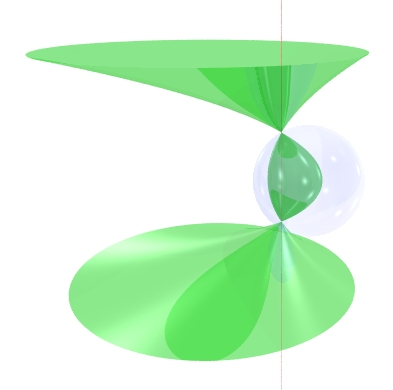In this picture, the line L does not pass through the center of the sphere, but meets it at two points, corresponding to c > 1 in the equation (1 - 2x + z2 - c2)2 - 4c2(x2 + y2) = 0. The horizontal cross-sections (by planes perpendicular to L) are ellipses, all with the same eccentricity (except at the singular points). Each cross-section by a plane containing L is a union of two parabolas.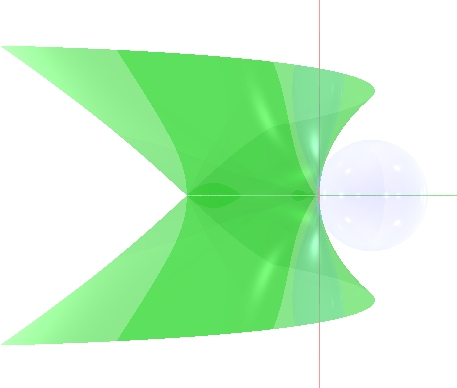This shows the line tangent to the sphere, corresponding to c = 1. The implicit equation simplifies to the quartic: z4 - 4xz2 - 4y2 = 0. The horizontal cross-sections are parabolas (except at the plane through the origin). Each cross-section by a plane containing L is a union of two parabolas tangent to L at the origin (except the plane containing C, which intersects the surface along the double line and one parabola). There are some solutions of the implicit equation which are not solutions of the equation b = |a - c|. Points on the negative x-axis (where the surface crosses itself) are equidistant from L and S, as are points on the line segment connecting the origin (0,0,0) to the center C = (1,0,0). However, points of the form (x,0,0), with x > 1, satisfy z4 - 4xz2 - 4y2 = 0, but are not equidistant from L and S. The shape can be parametrized by quadratic polynomials: x=u2-v2, y=2uv, z=2u, so it is a Steiner surface (of Type 4) and also falls into a classification of quadratically parametrized affine surfaces.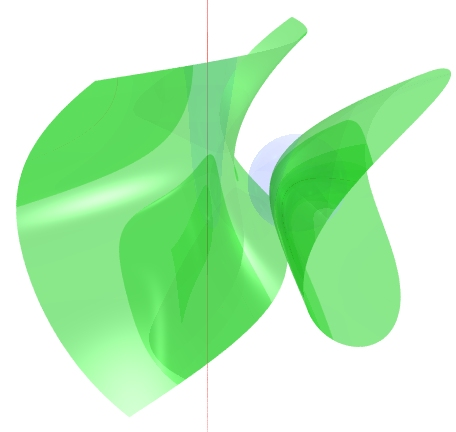The remaining case is that the sphere and line are disjoint, corresponding to 0 < c < 1. The surface has two components. The left half of the surface is the locus of equidistant points, which all lie outside the sphere, so the equation is b = a - c. Each of its horizontal cross-sections is one branch of a hyperbola. The constant c appearing in the implicit equation (1 - 2x + z2 - c2)2 - 4c2(x2 + y2) = 0. could change sign (replacing c with -c), without affecting the coefficients of the quartic. So, the solution set of the implicit equation includes not only the b = a - c locus, but also the b = a + c locus, where the distance to L is equal to the distance to the most distant point on S. This gives the right half of the surface, which cuts through the sphere. A horizontal cross-section of the whole surface gives both branches of a hyperbola.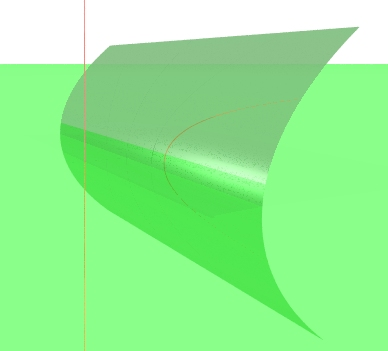There are two degenerate cases, the first being that the sphere could have radius c=0 and center C=(1,0,0). The surface becomes a parabolic cylinder (dark green), defined by the quadric equation 1 - 2x + z2 = 0. The second case is where c=0 and C=(0,0,0). The locus of points equidistant from L and S is the xy-plane, z = 0 (light green).

References
MR 55 #3978 K. Fladt and A. Baur, Analytische Geometrie spezieller Flächen und Raumkurven. Sammlung Vieweg, Band 136. Friedr. Vieweg & Sohn, Braunschweig, 1975.

These loci can also be considered as "line-sphere bisector" surfaces, in the sense of the following article:

M. Peternell, Geometric Properties of Bisector Surfaces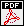, Graphical Models and Image Processing 62 (2000), 202-236.

Back to the graphics gallery.